# NCERT Solutions For Class 10 Maths Chapter 4 Quadratic Equations Ex 4.3

Get Free NCERT Solutions for Class 10 Maths Chapter 4 Ex 4.3 Quadratic Equations Class 10 Maths NCERT Solutions are extremely helpful while doing homework. Exercise 4.3 Class 10 Maths NCERT Solutions were prepared by Experienced ncert-books.in Teachers. Detailed answers of all the questions in Chapter 4 Maths Class 10 Quadratic Equations Exercise 4.3 Provided in NCERT Textbook

Topics and Sub Topics in Class 10 Maths Chapter 4 Quadratic Equations:

 Section Name Topic Name 4 Quadratic Equations 4.1 Introduction 4.2 Quadratic Equations 4.3 Solution of a Quadratic Equation by Factorisation 4.4 Solution of a Quadratic Equation by Completing the Square 4.5 Nature of Roots 4.6 Summary

You can also download the free PDF of Chapter 4 Ex 4.3 Quadratic Equations NCERT Solutions or save the solution images and take the print out to keep it handy for your exam preparation.

 Board CBSE Textbook NCERT Class Class 10 Subject Maths Chapter Chapter 4 Chapter Name Quadratic Equations Exercise Ex 4.3 Number of Questions Solved 11 Category NCERT Solutions

## NCERT Solutions For Class 10 Maths Chapter 4 Quadratic Equations Ex 4.3

NCERT Solutions for Class 10 Maths Chapter 4 Quadratic Equations Ex 4.3 are part of NCERT Solutions for Class 10 Maths. Here we have given NCERT Solutions for Class 10 Maths Chapter 4 Quadratic Equations Ex 4.3.

Question 1.
Find the roots of the following quadratic equations, if they exist, by the method of completing the square:
(i) 2x2 – 7x + 3 = 0
(ii) 2x2 + x – 4 = 0
(iii) 4x2 + 4√3x + 3 = 0
(iv) 2x2 + x + 4 = 0
Solution: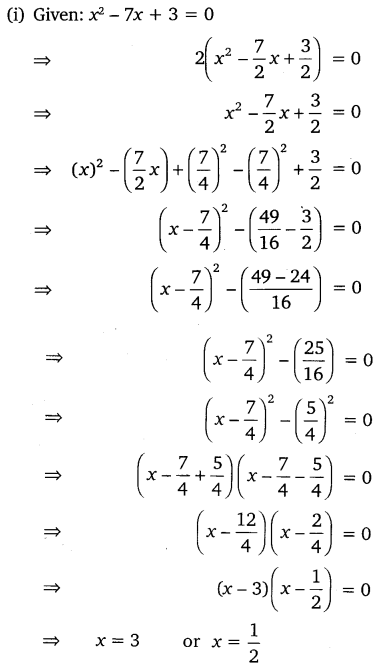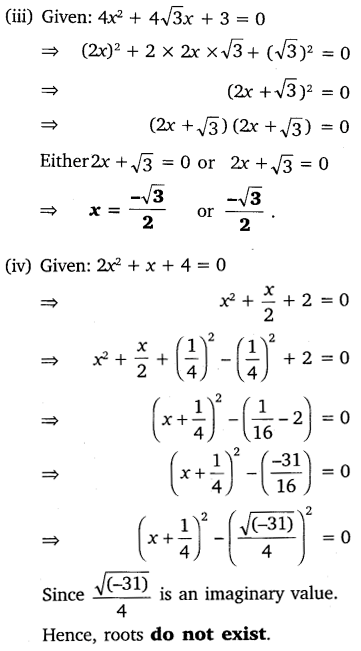Question 2.
Find the roots of the quadratic equations by applying the quadratic formula.
(i) 2x2 – 7x + 3 = 0
(ii) 2x2 – x + 4 = 0
(iii) 4x2 – 4√3x + 3 = 0
(iv) 2x2 – x + 4 = 0
Solution: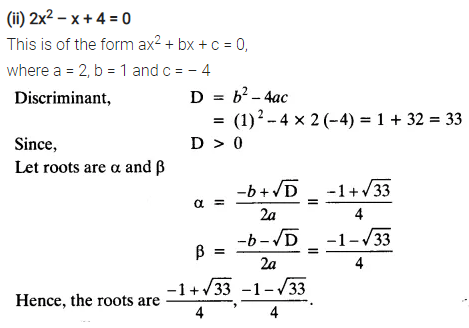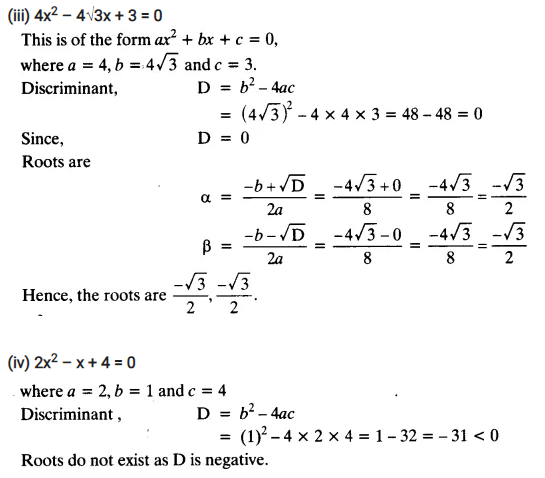Question 3.
Find the roots of the following equations:Solution:Question 4.
The sum of the reciprocals of Rehman’s ages, (in years) 3 years ago and 5 years from now is$\frac { 1 }{ 3 }$Find his present age.
Solution:Question 5.
In a class test, the sum of Shefali’s marks in Mathematics and English is 30. Had she got 2 marks more in Mathematics and 3 marks less in English, the product of their marks would have been 210. Find her marks in the two subjects.
Solution:Question 6.
The diagonal of a rectangular field is 60 metres more than the shorter side. If the longer side is 30 metres more than the shorter side, find the sides of the field.
Solution: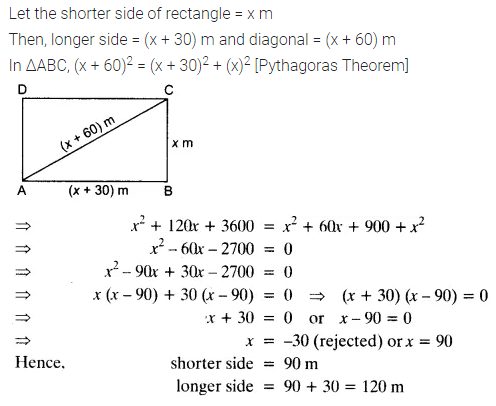Question 7.
The difference of squares of two numbers is 180. The square of the smaller number is 8 times the larger number. Find the two numbers.
Solution: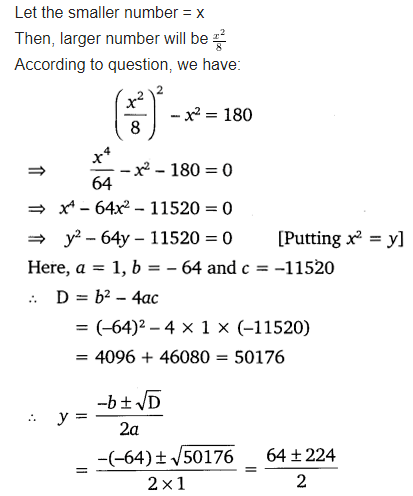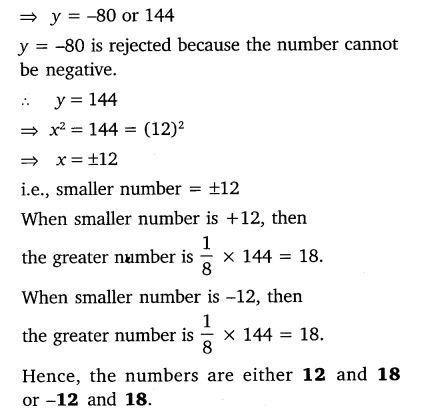Question 8.
A train travels 360 km at a uniform speed. If the speed had been 5 km/h more, it would have taken 1 hour less for the same journey. Find the speed of the train.
Solution: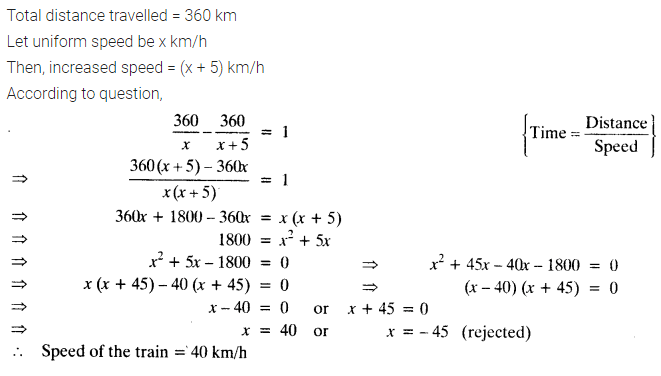Question 9.
Two water taps together can fill a tank in 9$\frac { 3 }{ 8 }$hours. The tap of larger diameter takes 10 hours less than the smaller one to fill the tank separately. Find the time in which each tap can separately fill the tank.
Solution: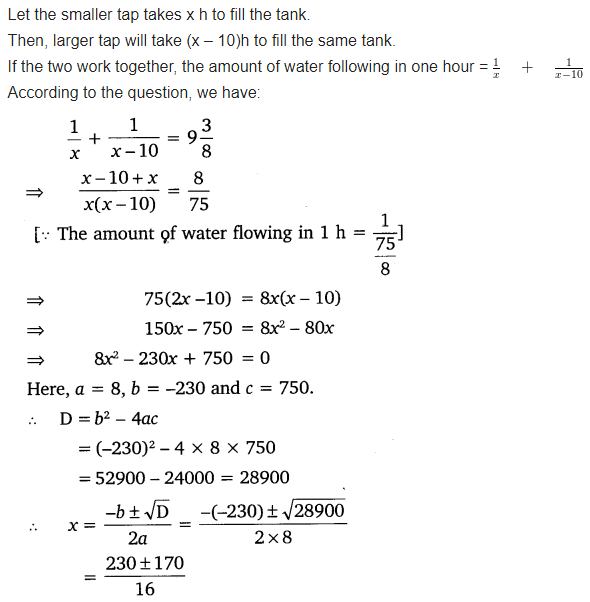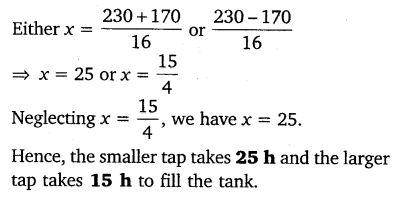Question 10.
An express train takes 1 hour less than a passenger train to travel 132 km between Mysore and Bengaluru (without taking into consideration the time they stop at intermediate stations). If the average speed of the express train is 11 km/h more than that of the passenger train, find the average speed of the two trains.
Solution: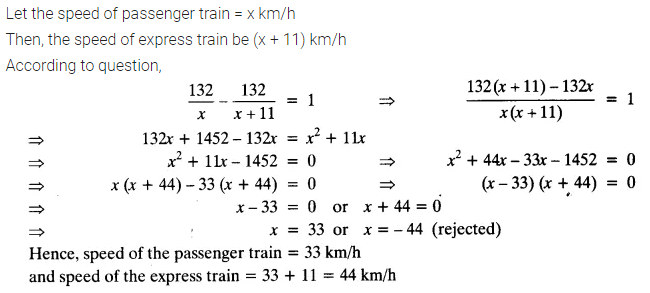Question 11.
Sum of the areas of two squares is 468 m2. If the difference of their perimeters is 24 m, find the sides of the two squares.
Solution: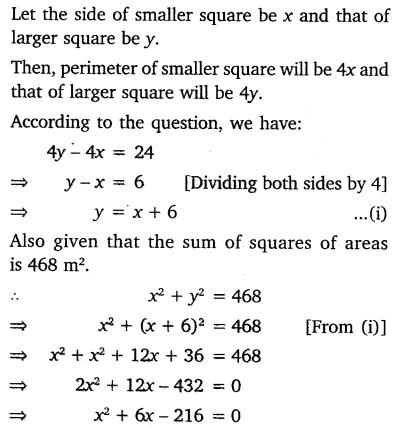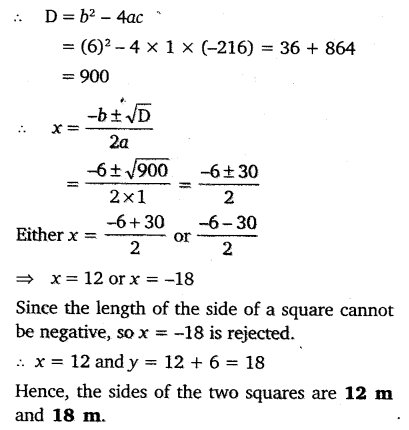error: Content is protected !!
+